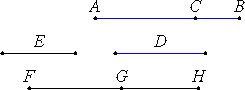# Proposition 50

To find the third binomial line.

Set out two numbers AC and CB such that the sum of them AB has to BC the ratio which a square number has to a square number, but does not have to AC the ratio which a square number has to a square number.

Also set out any other number D, not square, and let it not have to either of the numbers BA and AC the ratio which a square number has to a square number.

Set out any rational straight line E, and let it be contrived that D is to AB as the square on E is to the square on FG. Then the square on E is commensurable with the square on FG.X.9

And E is rational, therefore FG is also rational. And, since D does not have to AB the ratio which a square number has to a square number, neither does the square on E have to the square on FG the ratio which a square number has to a square number, therefore E is incommensurable in length with FG.

Next let it be contrived that the number BA is to AC as the square on FG is to the square on GH. Then the square on FG is commensurable with the square on GH.

X.9

But FG is rational, therefore GH is also rational. And, since BA does not have to AC the ratio which a square number has to a square number, neither does the square on FG have to the square on HG the ratio which a square number has to a square number, therefore FG is incommensurable in length with GH.

X.36

Therefore FG and GH are rational straight lines commensurable in square only. Therefore FH is binomial.

I say next that it is also a third binomial straight line.

V.22

Since D is to AB as the square on E is to the square on FG, and BA is to AC as the square on FG is to the square on GH, therefore, ex aequali, D is to AC as the square on E is to the square on GH.

X.9

But D does not have to AC the ratio which a square number has to a square number, therefore neither does the square on E have to the square on GH the ratio which a square number has to a square number. Therefore E is incommensurable in length with GH.

Since BA is to AC as the square on FG is to the square on GH, therefore the square on FG is greater than the square on GH.

V.19,Cor.

Let then the sum of the squares on GH and K equal the square on FG. Then, in conversion, AB is to BC as the square on FG is to the square on K.

X.9

But AB has to BC the ratio which a square number has to a square number, therefore the square on FG also has to the square on K the ratio which a square number has to a square number. Therefore FG is commensurable in length with K.

Therefore the square on FG is greater than the square on GH by the square on a straight line commensurable with FG.

And FG and GH are rational straight lines commensurable in square only, and neither of them is commensurable in length with E.

Therefore FH is a third binomial straight line.

Q.E.D.

## Guide

This proposition is not used in the rest of the Elements.